Short Theoretical Introduction to PIPSA
(Protein Interaction Property Similarity Analysis)

Detailed theory can be found in publications Blomberg etal, Proteins, 1998 and Wade etal, Intl. J. Quant. Chem. 2001 .

Molecular electrostatic potentials can be compared quantitatively by calculating similarity indices. Similarity indices have been developed for, and are usually applied to, the comparison of small molecules. They are, however, also useful for the analysis of macromolecules, allowing classification as well as prediction of the functional properties and active sites of macromolecules.  The Hodgkin index is commonly used to measure the similarity of two molecular potentials. It detects differences in sign, magnitude, and spatial behavior in the potentials and is given by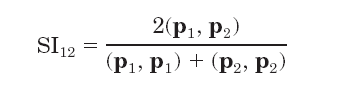where (p1, p2), (p1, p1), and (p2, p2) are the scalar products of the electrostatic potentials over the region where the potentials are compared.  Thus, SI12 = +1 if the two potentials are identical; SI12 = 0 if they are fully uncorrelated; SI12= -1 if they are anti-correlated.

If the electrostatic potentials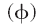are computed on a three-dimensional grid over the two superimposed molecules, the scalar product is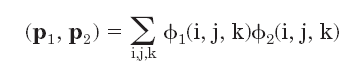where the summation is carried out over the grid points (i, j, k) that are within the region of interest.  The regions are usually chosen to represent a "skin" around the molecules having thickness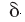. The "skin" of each molecule is defined as the volume remaining after excluding the region inside the surface accessible to the center of a probe with radius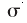, and the region outside the surface accessible to a probe of radius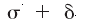. The "region of interest" is the intersection of the   "skins" of the two molecules.

The SI analysis can be performed for the complete molecular skins or restricted to particular chosen regions of the skins, e.g. around a protein binding site. In the latter case, a vector can be chosen pointing from the center of the proteins to the region to be studied.  The figure shows a blue copper protein (the copper ion represented by a sphere) with the definition of the conical region, where the potentials are compared: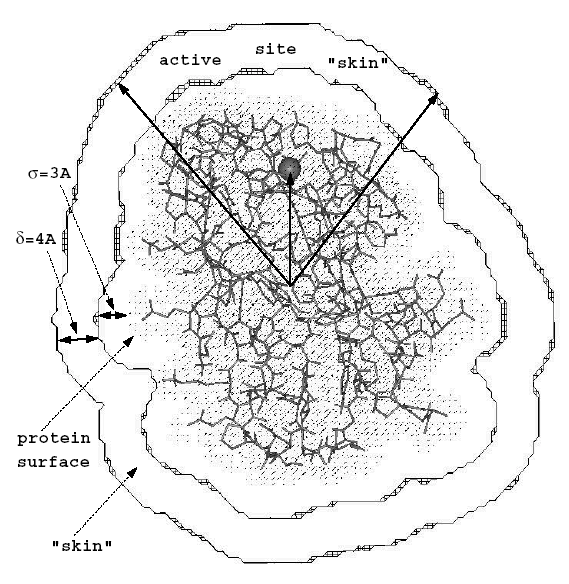Instead of comparing protein electrostatic potentials on a grid, SIs for electrostatic potentials can be computed using a simple analytical expression accounting for monopole and dipole terms: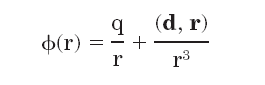then (so called "analytical") estimate of the electrostatic potential similarity index for 2 molecules is: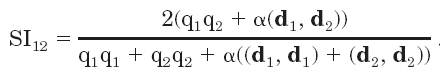with the parameter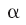depending on the region, where the potentials should be compared.
Interaction field similarity measure is not correlated with the sequence conservation measure:  large variety of interaction fields can be observed in a set of homologous proteins, as this can be seen from the comparison for all pairs of PH domain models of the electrostatic SI with the fraction of identical residues in each pair of sequences: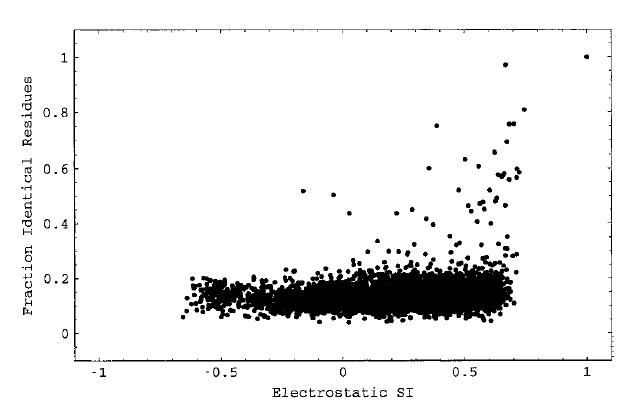[Back to Index]

Privacy Imprint